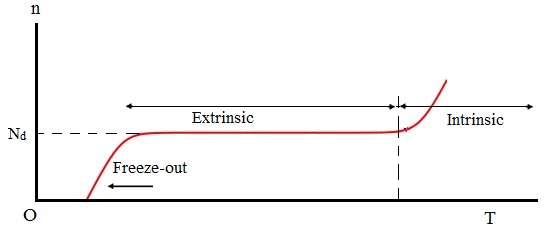#### Semiconductor Statistics, Physics tutorial

Definition:

Semiconductor statistics:

Semiconductors generally have both donors and acceptors. The electrons in the Conduction band can be made either through thermal excitation or by thermal ionization of the donors. Holes in the Valence band (VB) might be produced by interband excitation or through thermal excitation of electrons from the Valence band (VB) into the acceptor level. And in addition, electrons might fall from the donor levels to the acceptor level. The figure given below point out these different methods.Determining the concentrations of carriers, both electrons and holes, taking all such methods into account, is quite complex. We should treat some special cases, which are frequently encountered in practice. Two areas might be differentiated based on the physical parameters comprised: The intrinsic and the extrinsic regions.

The intrinsic region:

The concentration of carriers in the intrinsic region is found out by thermally induced interband transitions. In this region n = p. The intrinsic region obtains when the impurity doping is small. If we represent the concentrations of donors and acceptors by Nd and Na, the requirement for the validity of the intrinsic condition is:

n >> Nd, Na

As 'n' increases rapidly with temperature, the intrinsic condition becomes more favorable at higher temperatures. All the semiconductors, however, become intrinsic at adequately high temperatures (that is, unless the doping is oddly high).

The extrinsic region:

Quite often the intrinsic condition is not satisfied. For the general doping encountered, around 1015 cm-3, the number of carriers supplied through the impurities is large adequate to change the intrinsic concentration appreciably at room temperature. The contributions of impurities, however, frequently go beyond those carriers which are supplied by interband excitation. Whenever this is so, the sample is in the extrinsic region.

Two different kinds of extrinsic regions might be distinguished. The first takes place if the donor concentration greatly exceeds the acceptor concentration, that is, if Nd >> Na. In this case, the concentration of electrons might be computed quite readily. As the donor's ionization energy (that is, the binding energy) is quite small, all the donors are basically ionized, their electrons going into the Conduction band (CB). Thus, to a good approximation,

n = Nd

A semiconductor in which n >> p is termed as an n-type semiconductor ('n' for negative). Such a sample is characterized, as we have observed, via a great concentration of electrons.

The other kind of extrinsic region takes place if Na >> Nd, that is, the doping is mainly via acceptors. By using an argument identical to the above, one then consists of:

p = Na

That is, all the acceptors are ionized. Such a material is termed as a p-type semiconductor. This is characterized through a preponderance of holes.

In illustrating the ionization of donors (and acceptors), we supposed that the temperature is adequately high in such a way that all of these are ionized. This is certainly true at room temperature. However if the temperature is progressively lowered, a point is reached at which the thermal energy becomes too small to cause the electron excitation. In that condition, the electrons fall from the Conduction band into the donor level, and the conductivity of the sample reduces dramatically. This is termed to as freeze-out, in that the electrons are now 'frozen' at their impurity sites. The temperature at which freeze-out occurs is Ed ~ kT that provides a temperature of around 100°KThe variation of the electron concentration having temperature in an n-type sample is represented schematically in the above figure.

Tutorsglobe: A way to secure high grade in your curriculum (Online Tutoring)